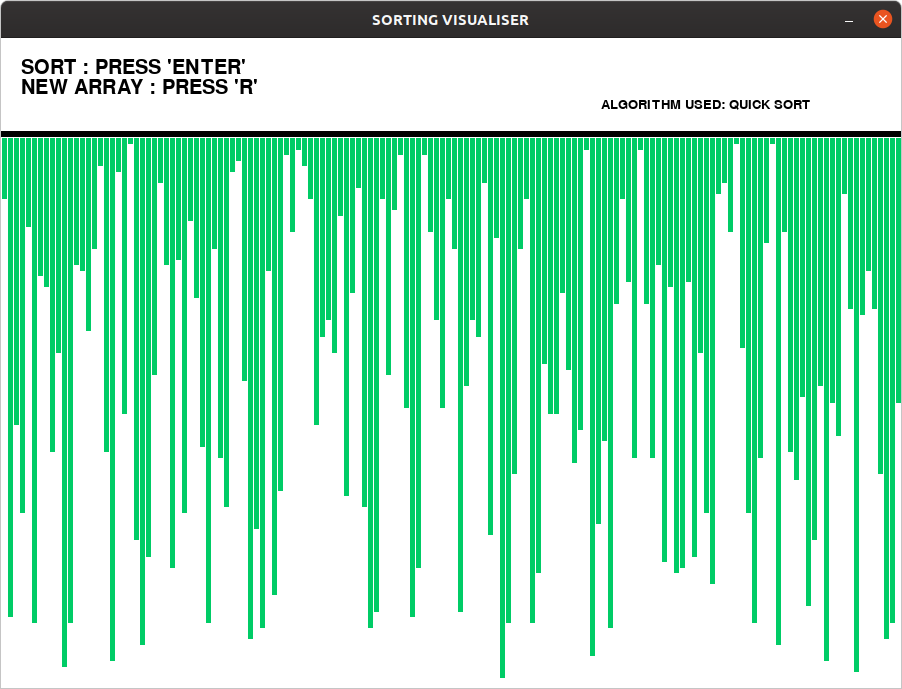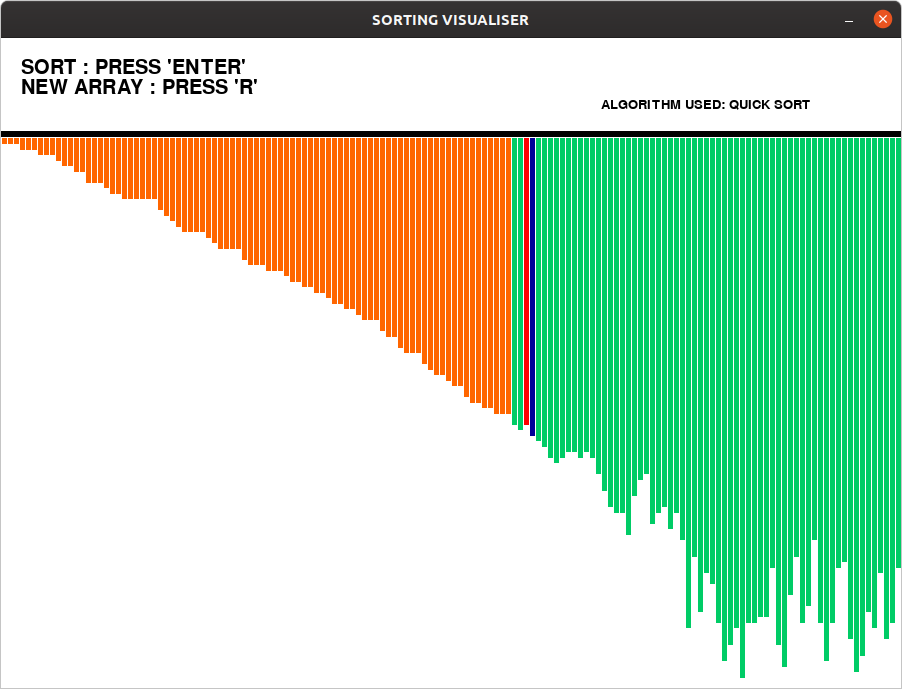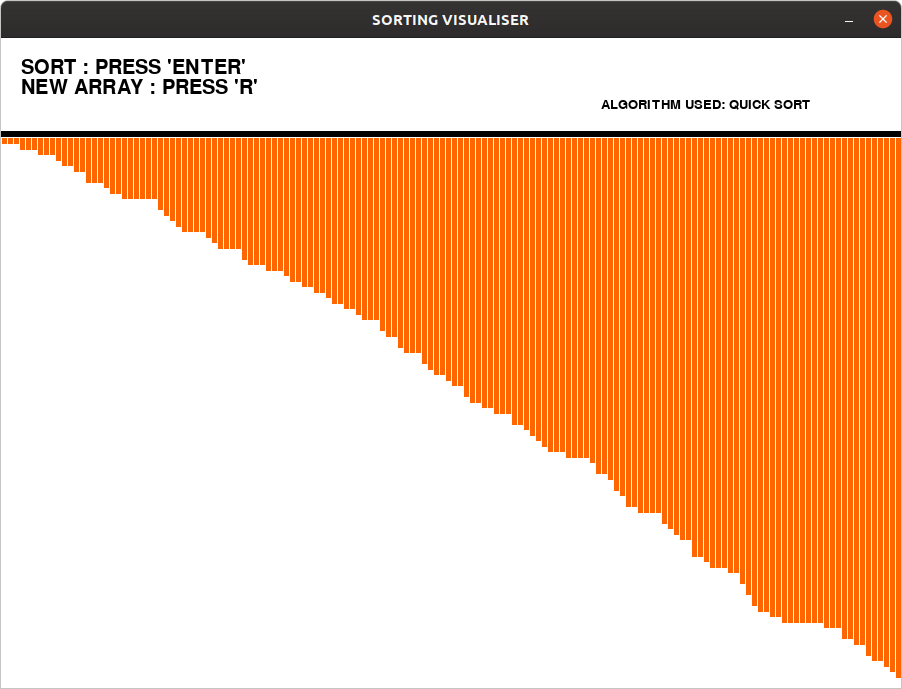# Sorting Algorithm Visualization : Quick Sort

An algorithm like Quicksort algorithm is hard to understand theoretically. We can understand easily by visualizing such kind of algorithms. In this article, a program that visualizes the Quicksort Algorithm has been implemented.
The Graphical User Interface(GUI) is implemented in python using pygame library.
Approach:

• An array of random values is generated and are drawn as lines(bars) in the window.
• Since the algorithm performs the operation very fast, pygame.time.delay() has been used to slow down the process.
• Assign specific keys for each operation (start sorting, reset bars).
• The actions are performed using `‘pygame.event.get()’` method, which stores all the events which user performs.
• Different colors are used to indicate types of bar.
• Green – Unsorted bar
• Blue – Pivot bar
• Orange – Sorted bar

Examples:

Input:
Press “Enter” key to Perform Visualization.
Press “r” key to generate new array.
Output:
Initial:Sorting:Final:Below is the implementation of the above visualizer:

## Python

 `# Python implementation of the  ` `# Sorting visualiser: Quick Sort ` ` `  `# Imports ` `import` `pygame ` `import` `random ` `pygame.font.init() ` ` `  `# Total window ` `screen ``=` `pygame.display.set_mode( ` `            ``(``900``, ``650``) ` `        ``) ` ` `  `# Title and Icon  ` `pygame.display.set_caption(``"SORTING VISUALISER"``) ` ` `  `# Uncomment below lines for setting  ` `# up the icon for the visuliser ` `# img = pygame.image.load('sort_icon.png') ` `# pygame.display.set_icon(img) ` ` `  `# Boolean variable to run  ` `# the program in while loop ` `run ``=` `True` ` `  `# Window size and some initials ` `width ``=` `900` `length ``=` `600` `array ``=``[``0``]``*``151` `arr_clr ``=``[(``0``, ``204``, ``102``)]``*``151` `clr_ind ``=` `0` `clr ``=``[(``0``, ``204``, ``102``), (``255``, ``0``, ``0``),\ ` `      ``(``0``, ``0``, ``153``), (``255``, ``102``, ``0``)] ` `fnt ``=` `pygame.font.SysFont(``"comicsans"``, ``30``) ` `fnt1 ``=` `pygame.font.SysFont(``"comicsans"``, ``20``) ` ` `  ` `  `# Function to generate new Array ` `def` `generate_arr(): ` `    ``for` `i ``in` `range``(``1``, ``151``): ` `        ``arr_clr[i]``=` `clr[``0``] ` `        ``array[i]``=` `random.randrange(``1``, ``100``) ` `         `  `# Intially generate a array ` `generate_arr()  ` ` `  `# Function to refill the  ` `# updates on the window ` `def` `refill(): ` `    ``screen.fill((``255``, ``255``, ``255``)) ` `    ``draw() ` `    ``pygame.display.update() ` `    ``pygame.time.delay(``30``) ` `     `  `# Sorting Algo:Quick sort ` `def` `quicksort(array, l, r): ` `    ``if` `l

Output:

```
```

Attention reader! Don’t stop learning now. Get hold of all the important DSA concepts with the DSA Self Paced Course at a student-friendly price and become industry ready.

My Personal Notes arrow_drop_upCheck out this Author's contributed articles.

If you like GeeksforGeeks and would like to contribute, you can also write an article using contribute.geeksforgeeks.org or mail your article to contribute@geeksforgeeks.org. See your article appearing on the GeeksforGeeks main page and help other Geeks.

Please Improve this article if you find anything incorrect by clicking on the "Improve Article" button below.